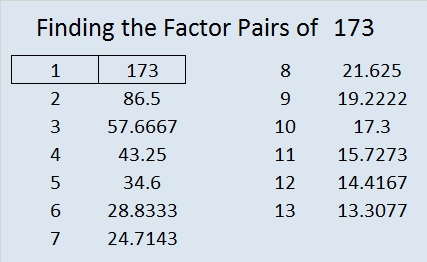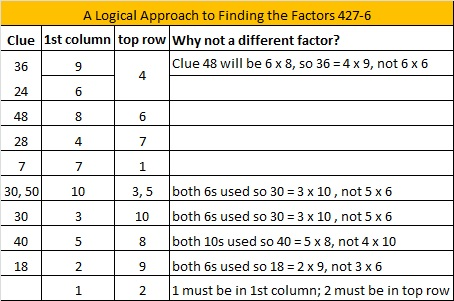# 173 and Level 6

• 173 is a prime number.
• Prime factorization: 173 is prime.
• The exponent of prime number 173 is 1. Adding 1 to that exponent we get (1 + 1) = 2. Therefore 173 has exactly 2 factors.
• Factors of 173: 1, 173
• Factor pairs: 173 = 1 x 173
• 173 has no square factors that allow its square root to be simplified. √173 ≈ 13.1529How do we know that 173 is a prime number? If 173 were not a prime number, then it would be divisible by at least one prime number less than or equal to √173 ≈ 13.2. Since 173 cannot be divided evenly by 2, 3, 5, 7, 11, or 13, we know that 173 is a prime number.Excel file of puzzles and previous week’s factor solutions: 10 Factors 2014-07-07